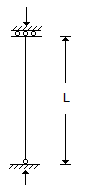# Civil Engineering - UPSC Civil Service Exam Questions

21.

In a situation where torsion is dominant, which one of the following is the desirable section ?

 A. Angle section B. Channel section C. I-section D. Box type section

Explanation:

No answer description available for this question. Let us discuss.

22.

Consider the following statements :
(a) Setting and hardening of cement takes place after the addition of water.
(b) Water causes hydration and hydrolysis of the constituent compounds of cement which act as binders
Which of the statements given above is/are correct ?

 A. 1 only B. 2 only C. Both 1 and 2 D. Neither 1 nor 2

Explanation:

No answer description available for this question. Let us discuss.

23.

The effective length of the member shown in the given figure is equal toA. 1.2 L B. 1.5 L C. 2.0 L D. 3.0 L

Explanation:

No answer description available for this question. Let us discuss.

24.

A surface longitudinally centered on the extended runway centre line and extending outward and upward from each end of the primary surface is known as

 A. conical surface B. transition surface C. approach surface D. horizontal surface

Explanation:

No answer description available for this question. Let us discuss.

25.

A flownet for an earth dam on impervious foundation consists of 4 flow channels and 15 equipotential drops. The full reservoir level is 15 m above the downstream horizontal filter. Given that horizontal permeability is 9 x 10-6 m/s and vertical permeability is 1 x 10-6 m/s, the quantity of seepage through the dam will be

 A. 36 ml/s/m B. 25 ml/s/m C. 20 ml/s/m D. 15 ml/s/m